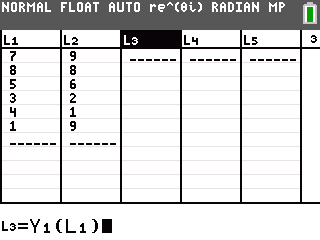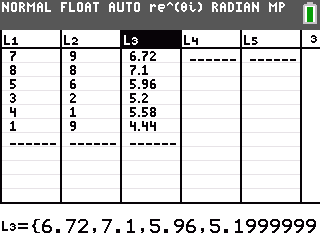# Knowledge Base

## Solution 34575: Calculating Y-Hat Values on the TI-84 Plus Family of Graphing Calculators.

### How do I calculate y-hat values using the on the TI-84 Plus family of graphing calculators?

After finding the regression equation for a data set, it is helpful to know what y-value the regression equation would predict for any x-value from the data set. This corresponding y-value is denoted y-hat. Y-hat values are calculated by substituting the x-values from the data set into the regression equation.

To calculate y-hat values for a data set follow the example below:

Please Note: The TI-84 Plus and TI-84 Plus Silver Edition require Operating System (OS) v 2.55MP to have access to the stat wizard view below. To verify what OS is installed press [2nd] followed by [+] then in the menu that appears select [1:About]. If you do not have this version of OS please upgrade to this version.

Data for this example:

L1: {7,8,5,3,4,1}
L2: {9,8,6,2,1,9}

• Press [STAT]  to access the STAT list editor.
• Input the data in the L1 and L2 lists, making sure to press [ENTER] after each entry.
• Press [2nd] [MODE] to QUIT and return to the home screen.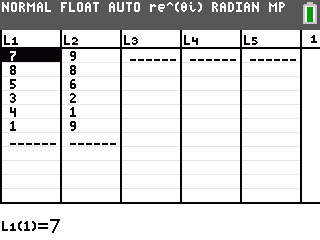Calculating and storing the regression equation:

• Press [STAT], arrow right to highlight CALC and press  to select the LinReg(ax+b) function.
• Leave L1 for Xlist and L2 for Ylist.
• Arrow down to Store RegEQ: and press [VARS].
• Arrow right to highlight Y-VARS and press  to select the Y1 function.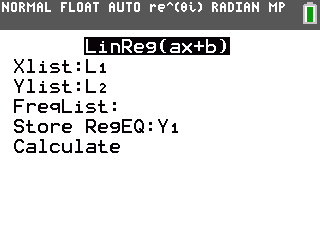• Arrow down to Calculate and press [ENTER].
• The linear regression data will be displayed. The regression equation will also be stored to Y1.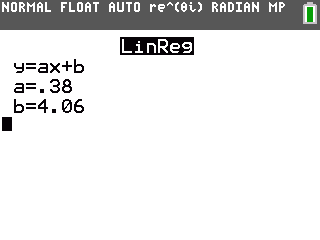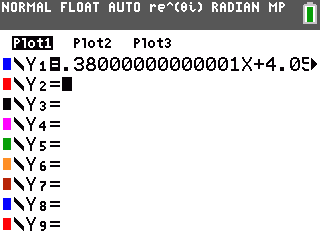Calculating y-hat values:

• Press [STAT]  to access the STAT list editor.
• Scroll over to L3, scroll up to highlight L3 and press [ENTER] to input a formula for the L3 list.
• Press [VARS], arrow right to highlight Y-VARS and press  to select the Y1 function.
• Press [ ( ] [2nd] [L1] [ ) ]. Press [ENTER] to calculate the y-hat values which will be displayed in L3.